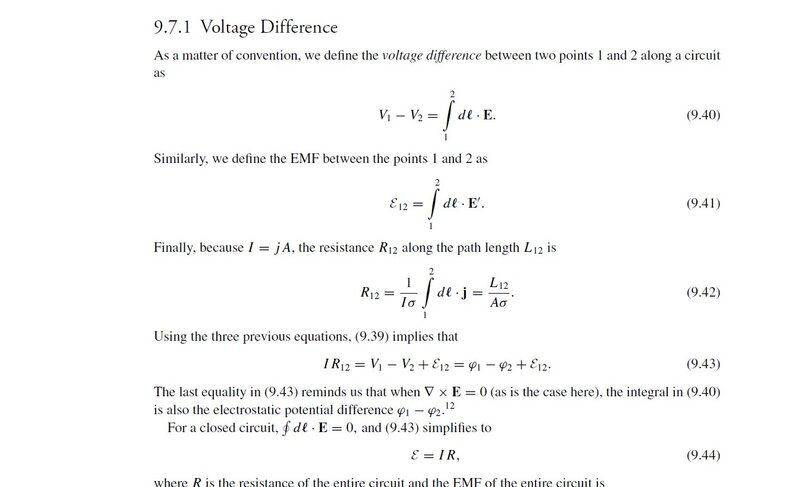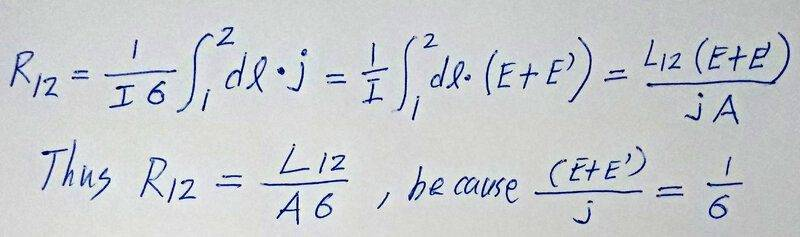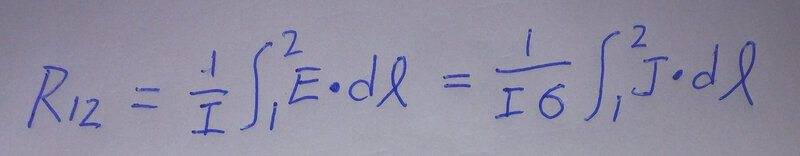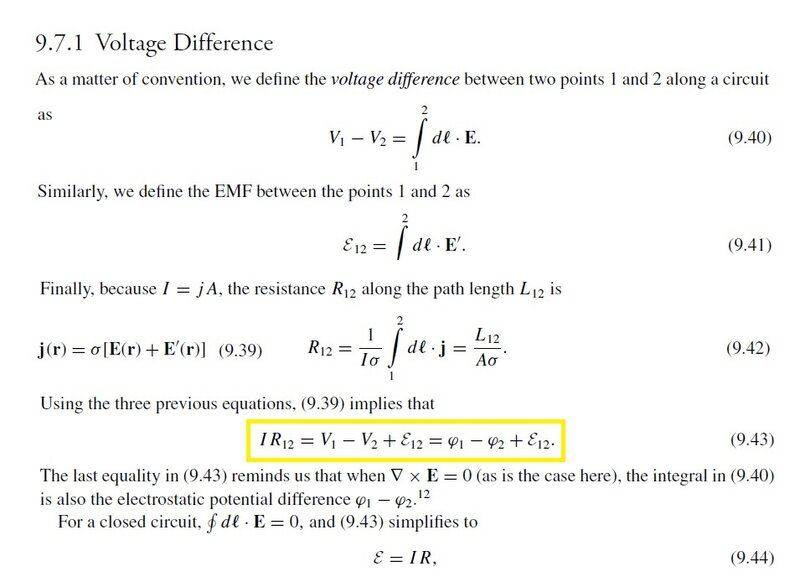# Deriving expression for resistance in terms of current density

• I
spindecide
Is there a way to obtain equation 9.42 (I is current, j is current density, and sigma is conductivity) in the following image (from Modern Electrodynamics by Andrew Zangwill, the part on electromotive force) besides using V=IR and substituting the line integral of j/conductivity for V? The aforementioned way requires that j=conductivity*E, but due to the emf, j should be equal to conductivity*[E + E'], where E' is a fictitious electric field representing the effect of any source of EMF.alan123hk
I think this equation is reasonable and I don't see any problem with it.spindecide
I think this equation is reasonable and I don't see any problem with it.

But how is R written as the first integral?

alan123hk
But how is R written as the first integral?
My personal opinion as follow.spindecide
But j is not just equal to sigma times E as the effect of the emf needs to be considered, so that j equals sigma times (E + E').

alan123hk
A very useful equation is shown here$$V_1-V_2=IR_{12}-\varepsilon _{12}~~~~~\Rightarrow ~~~~~V_1-V_2=IR_{12}-\int_1^2~dl\cdot~E^{'}$$ That is to say, the voltage difference in a circuit with EMF is not equal to IR, but equal to IR minus EMF. This is actually an equation very familiar and commonly used by electrical and electronics engineers when conducting circuit analysis.

It is also worth noting that $$IR_{12}=(V_1-V_2)+\varepsilon _{12}=\int_1^2~dl\cdot~(E+E^{'}),~~~~\text{so}~~R=\frac {1}{I} \int_1^2~dl\cdot~(E+E^{'})$$
Everything is in perfect harmony, without contradictions.Last edited:
alan123hk
But j is not just equal to sigma times E as the effect of the emf needs to be considered, so that j equals sigma times (E + E').
You are right, I accidentally wrote the equation wrong.

••